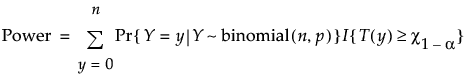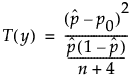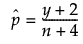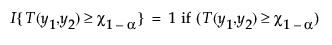Design of Experiments Guide > Prospective Sample Size and Power > One Sample Proportion Calculator > Statistical Details for the One Proportion Calculator
Publication date: 08/13/2020

## Statistical Details for the One Proportion Calculator

The one proportion sample size computations use exact methods based on the binomial distribution. Exact calculations guarantee that the stated power level is obtained.

### Agresti-Coull Method

The exact Agresti-Coull method uses the adjusted Wald-based test statistic. JMP calculates power under the two-sided null hypothesis as follows:where:and 0 otherwise, and

χ1-α is the (1 - α)thquantile of the χ12 distribution.

Because there is not a closed-form expression for n or p0, numerical techniques are used to solve for n or p0.